This article uses an example to introduce to genetic algorithms (GAs) for optimization. It discusses two operators (mutation and crossover) that are important in implementing a genetic algorithm. It discusses choices that you must make when you implement these operations.

Some programmers love using genetic algorithms. Genetic algorithms are heuristic methods that can be used to solve problems that are difficult to solve by using standard discrete or calculus-based optimization methods. A genetic algorithm tries to mimic natural selection and evolution by starting with a population of random candidates. Candidates are evaluated for "fitness" by plugging them into the objective function. The better candidates are combined to create a new set of candidates. Some of the new candidates experience mutations. Eventually, over many generations, a GA can produce candidates that approximately solve the optimization problem. Randomness plays an important role. Re-running a GA with a different random number seed might produce a different solution.

Critics of genetic algorithms note two weaknesses of the method. First, you are not guaranteed to get the optimal solution. However, in practice, GAs often find an acceptable solution that is good enough to be used. The second complaint is that the user must make many heuristic choices about how to implement the GA. Critics correctly note that implementing a genetic algorithm is as much an art as it is a science. You must choose values for hyperparameters and define operators that are often based on a "feeling" that these choices might result in an acceptable solution.

This article discusses two fundamental parts of a genetic algorithm: the crossover and the mutation operators. The operations are discussed by using the binary knapsack problem as an example. In the knapsack problem, a knapsack can hold W kilograms. There are N objects, each with a different value and weight. You want to maximize the value of the objects you put into the knapsack without exceeding the weight. A solution to the knapsack problem is a 0/1 binary vector b. If b[i]=1, the i_th object is in the knapsack; if b[i]=0, it is not.

### A brief overview of genetic algorithms

The SAS/IML User's Guide provides an overview of genetic algorithms. The main steps in a genetic algorithm are as follows:

• Encoding: Each potential solution is represented as a chromosome, which is a vector of values. The values can be binary, integer-valued, or real-valued. (The values are sometimes called genes.) For the knapsack problem, each chromosome is an N-dimensional vector of binary values.
• Fitness: Choose a function to assess the fitness of each candidate chromosome. This is usually the objective function for unconstrained problems, or a penalized objective function for problems that have constraints. The fitness of a candidate determines the probability that it will contribute its genes to the next generation of candidates.
• Selection: Choose which candidates become parents to the next generation of candidates.
• Crossover (Reproduction): Choose how to produce children from parents.
• Mutation: Choose how to randomly mutate some children to introduce additional diversity.

### The mutation operator

The mutation operator is the easiest operation to understand. In each generation, some candidates are randomly perturbed. By chance, some of the mutations might be beneficial and make the candidate more fit. Others are detrimental and make the candidate less fit.

For a binary chromosome, a mutation consists of changing the parity of some proportion of the elements. The simplest mutation operation is to always change k random elements for some hyperparameter k < N. A more realistic mutation operation is to choose the number of sites randomly according to a binomial probability distribution with hyperparameter pmut. Then k is a random variable that differs for each mutation operation.

The following SAS/IML program chooses pmut=0.2 and defines a subroutine that mutates a binary vector, b. In this example, there are N=17 items that you can put into the knapsack. The subroutine first uses the Binom(pmut, N) probability distribution to obtain a random number of sites, k, to mutate. (But if the distribution returns 0, set k=1.) The SAMPLE function then draws k random positions (without replacement), and the values in those positions are changed.

```proc iml; call randseed(12345); N = 17; /* size of binary vector */ ProbMut= 0.2; /* mutation in 20% of sites */   /* Mutation operator for a binary vector, b. The number of mutation sites k ~ Binom(ProbMut, N), but not less than 1. Randomly sample (without replacement) k sites. If an item is not in knapsack, put it in; if an item is in the sack, take it out. */ start Mutate(b) global(ProbMut); N = nrow(b); k = max(1, randfun(1, "Binomial", ProbMut, N)); /* how many sites? */ j = sample(1:N, k, "NoReplace"); /* choose random elements */ b[j] = ^b[j]; /* mutate these sites */ finish;   Items = 5:12; /* choose items 5-12 */ b = j(N,1,0); b[Items] = 1; bOrig = b; run Mutate(b); print (bOrig`)[L="Original b" c=(1:N)] (b`)[L="Randomly Mutated b" c=(1:N)];```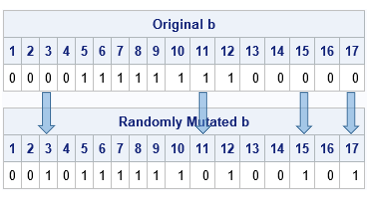In this example, the original chromosome has a 1 in locations 5:12. The binomial distribution randomly decides to mutate k=4 sites. The SAMPLE function randomly chooses the locations 3, 11, 15, and 17. The parity of those sites is changed. This is seen in the output, which shows that the parity of these four sites differs between the original and the mutated b vector.

Notice that you must choose HOW the mutation operator works, and you must choose a hyperparameter that determines how many sites get mutated. The best choices depend on the problem you are trying to solve. Typically, you should choose a small value for the probability pmut so that only a few sites are mutated.

In the SAS/IML language, there are several built-in mutation operations that you can use. They are discussed in the documentation for the GASETMUT subroutine.

### The crossover operator

The crossover operator is analogous to the creation of offspring through sexual reproduction. You, as the programmer, must decide how the parent chromosomes, p1 and p2, will combine to create two children, c1 and c2. There are many choices you can make. Some reasonable choices include:

• Randomly choose a location s, 1 ≤ s ≤ N. You then split the parent chromosomes at that location and exchange and combine the left and right portions of the parents' chromosomes. One child chromosome is c1 = p1[1:s] // p2[s+1:N] and the other is c2 = p2[1:s] // p1[s+1:N]. Note that each child gets some values ("genes") from each parent.
• Randomly choose a location s, 1 ≤ s ≤ N. Divide the first chromosome into subvectors of length s and N-s. Divide the second chromosome into subvectors of length N-s and s. Exchange the subvectors of the same length to form the child chromosomes.
• Randomly choose k locations. Exchange the locations between parents to form the child chromosomes.

The following SAS/IML function implements the third crossover method. The method uses a hyperparameter, pcross, which is the probability that each location in the chromosome is selected. On average, about Npcross locations will be selected. In the following program, pcross = 0.3, so we expect 17(0.3)=5.1 values to be exchanged between the parent chromosomes to form the children:

```start uniform_cross(child1, child2, parent1, parent2) global(ProbCross); b = j(nrow(parent1), 1); call randgen(b, "Bernoulli", ProbCross); /* 0/1 vector */ idx = loc(b=1); /* locations to cross */   child1 = parent1; /* initialize children */ child2 = parent2; if ncol(idx)>0 then do; /* exchange values */ child1[idx] = parent2[idx]; /* child1 gets some from parent2 */ child2[idx] = parent1[idx]; /* child2 gets some from parent1 */ end; finish;   ProbCross = 0.3; /* crossover 25% of sites */ Items = 5:12; p1 = j(N,1,0); p1[Items] = 1; /* choose items 5-12 */ Items = 10:15; p2 = j(N,1,0); p2[Items] = 1; /* choose items 10-15 */ run uniform_cross(c1, c2, p1, p2); print (p1`)[L="Parent1" c=(1:N)], (p2`)[L="Parent2" c=(1:N)], (c1`)[L="Child1" c=(1:N)], (c2`)[L="Child2" c=(1:N)];```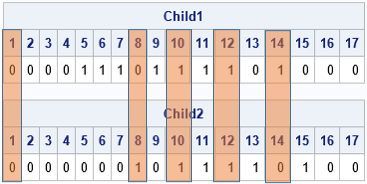I augmented the output to show how the child chromosomes are created from their parents. For this run, the selected locations are 1, 8, 10, 12, and 14. The first child gets all values from the first parent except for the values in these five positions, which are from the second parent. The second child is formed similarly.

When the parent chromosomes resemble each other, the children will resemble the parents. However, if the parent chromosomes are very different, the children might not look like either parent.

Notice that you must choose HOW the crossover operator works, and you must choose a hyperparameter that determines how to split the parent chromosomes. In more sophisticated crossover operations, there might be additional hyperparameters, such as the probability that a subchromosome from a parent gets reversed in the child. There are many heuristic choices to make, and the best choice is not knowable.

In the SAS/IML language, there are many built-in crossover operations that you can use. They are discussed in the documentation for the GASETCRO subroutine.

### Summary

Genetic algorithms can solve optimization problems that are intractable for traditional mathematical optimization algorithms. But the power comes at a cost. The user must make many heuristic choices about how the GA should work. The user must choose hyperparameters that control the probability that certain events happen during mutation and crossover operations. The algorithm uses random numbers to generate new potential solutions from previous candidates. This article used the SAS/IML language to discuss some of the choices that are required to implement these operations.

A subsequent article discusses how to implement a genetic algorithm in SAS.

The post Crossover and mutation: An introduction to two operations in genetic algorithms appeared first on The DO Loop.Photograph by Poussin Jean, license CC BY-SA 3.0, via Wikimedia Commons

The partition problem has many variations, but recently I encountered it as an interactive puzzle on a computer. (Try a similar game yourself!) The player is presented with an old-fashioned pan-balance scale and a set of objects of different weights. The challenge is to divide (or partition) the objects into two group. You put one group of weights on one side of the scale and the remaining group on the other side so that the scale balances.

Here's a canonical example of the partition problem for two groups. The weights of six items are X = {0.4, 1.0, 1.2, 1.7, 2.6, 2.7}. Divide the objects into two groups of three items so that each group contains half the weight, which is 4.8 for this example. Give it a try! I'll give a solution in the next section.

As is often the case, there are at least two ways to solve this problem: the brute-force approach and an optimization method that minimizes the difference in weights between the two groups. One advantage of the brute-force approach is that it is guaranteed to find all solutions. However, the brute-force method quickly becomes impractical as the number of items increases.

This article considers brute-force solutions. A more elegant solution will be discussed in a future article.

### Permutations: A brute-force approach

Let's assume that the problem specifies the number of items that should be placed in each group. One way to specify groups is to use a vector of ±1 values to encode the group to which each item belongs. For example, if there are six items, the vector c = {-1, -1, -1, +1, +1, +1} indicates that the first three items belong to one group and the last three items belong to the other group.

One way to solve the partition problem for two groups is to consider all permutations of the items. For example, Y = {0.4, 1.7, 2.7, 1.0, 1.2, 2.6} is a permutation of the six weights in the previous section. The vector c = {-1, -1, -1, +1, +1, +1} indicates that the items {0.4, 1.7, 2.7} belong to one group and the items {1.0, 1.2, 2.6} belong to the other group. Both groups have 4.8 units of weight, so Y is a solution to the partition problem.

Notice that the inner product Y`*c = 0 for this permutation. Because c is a vector of ±1 values, the inner product is the difference between the sum of weights in the first group and the sum of weights in the second group. An inner product of 0 means that the sums are the same in both groups.

### A program to generate all partitions

Let's see how you can use the ALLPERM function in SAS/IML to solve the two-group partition problem. Since the number of permutations grows very fast, let's use an example that contains only four items. The weights of the four items are X = {1.2, 1.7, 2.6, 3.1}. We want two items in each group, so define c = {-1, -1, 1, 1}. We search for a permutation Y = π(X), such that Y`*c = 0. The following SAS/IML program generates ALL permutations of the integers {1,2,3,4}. For each permutation, the sum of the first two weights is compared to the sum of the last two weights. The permutations for which Y`*c = 0 are solutions to the partition problem.

```proc iml; X = {1.2, 1.7, 2.6, 3.1}; c = { -1, -1, 1, 1}; /* k = 2 */   /* Brute Force Method 1: Generate all permutations of items */ N = nrow(X); P = allperm(N); /* all permutations of 1:N */ Y = shape( X[P], nrow(P), ncol(P) ); /* reshape to N x N! matrix */ z = Y * c; /* want to find z=0 (or could minimize z) */ idx = loc(abs(z) < 1E-8); /* indices where z=0 in finite precision */ Soln = Y[idx, ]; /* return all solutions; each row is solution */```

This program generates P, a matrix whose rows contain all permutations of four elements. The matrix Y is a matrix where each row is a permutation of the weights. Therefore, Y*c is the vector of all differences. When a difference is zero, the two groups contain the same weights. The following statements count how many solutions are found and print the first solution:

```numSolns = nrow(soln); s = soln[1,]; print numSolns, s[c={'L1' 'L2' 'R1' 'R2'}];```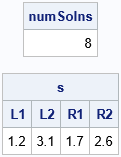There are a total of eight solutions. One solution is to put the weights {1.2, 3.1} in one group and the weights {1.7, 2.6} in the other group. What are the other solutions? For this set of weights, the other solutions are trivial variations of the first solution. The following statement prints all the solutions:

`print soln[c={'L1' 'L2' 'R1' 'R2'}];`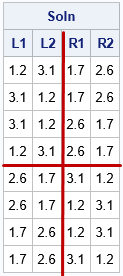I have augmented the output so that it is easier to see the structure. In the first four rows, the values {1.2, 3.1} are in the first group and the values {1.7, 2.6} are in the second group. In the last four rows, the values switch groups. Thus, this method, which is based on generating all permutations, generates a lot of solutions that are qualitatively the same, in practice.

### Combinations: Another brute-force approach

The all-permutation method generates N! possible partitions and, as we have seen, not all the partitions are qualitatively different. Thus, using all permutations is inefficient. A more efficient (but still brute-force) method is to use combinations instead of permutations. Combinations are essentially a sorted version of a permutation. The values {1, 2, 3}, {2, 1, 3}, and {3, 2, 1} are different permutations, whereas there is only one combination ({1, 2, 3}) that contains these three numbers. If there are six items and you want three items in each group, there are 6! = 720 permutations to consider, but only "6 choose 3" = 20 combinations.

The following SAS/IML function uses combinations to implement a brute-force solution of the two-group partition problem. The Partition2_Comb function takes a vector of item weights and a vector (nPart) that contains the number of items that you want in the first and second groups. If you want k items in the first group, the ALLCOMB function creates the complete set of combinations of k indices. The SETDIFF function computes the complementary set of indices. For example, if there are six items and {1, 4, 5} is a set of indices, then {2, 3, 6} is the complementary set of indices. After the various combinations are defined, the equation Y*c = 0 is used to find solutions, if any exist, where the first k elements of c are -1 and the last N-k elements are +1.

```/* Brute Force Method 2: Generate all combination of size k, N-k */ start Partition2_Comb(_x, k, tol=1e-8); x = colvec(_x); N = nrow(x); call sort(x); /* Optional: standardize the output */   c = j(k, 1, -1) // j(N-k, 1, 1); /* construct +/-1 vector */ L = allcomb(N, k); /* "N choose k" possible candidates in "left" group */ R = j(nrow(L), N-k, .); do i = 1 to nrow(L); R[i,] = setdif(1:N, L[i,]); /* complement indices in "right" group */ end; P = L || R; /* combine the left and right indices */   Y = shape( X[P], nrow(P) ); /* reshape X[P] into an N x (N choose k) matrix */ z = Y * c; /* want to find z=0 (or could minimize z) */ solnIdx = loc(abs(z) < tol); /* indices where z=0 in finite precision */ if ncol(solnIdx) = 0 then soln = j(1, N, .); /* no solution */ else soln = Y[solnIdx, ]; /* each row is solution */ return soln; /* return all solutions */ finish;   /* test the function on a set that has 11 items, partitioned into groups of 5 and 6 */ x = {0.8, 1.2, 1.3, 1.4, 1.6, 1.8, 1.9, 2.0, 2.2, 2.3, 2.5}; soln = Partition2_Comb(x, 5); numSolns = nrow(soln); print numSolns, soln[c=(('L1':'L5') || ('R1':'R6'))];```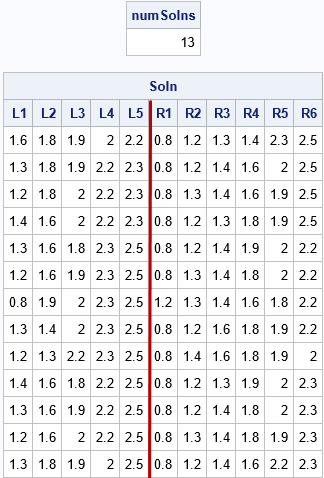The output shows 13 solutions for a set of 11 items that are partitioned into two groups, one with five items and the other with 11-5=6 items. For the solution, each partition has 9.5 units of weight.

### Summary

This article shows some SAS/IML programming techniques for using a brute-force method to solve the partition problem on N items. In the partition problem, you split items into two groups that have k and N-k items so that the weight of the items in each group is equal. This article introduced the solution in terms of all permutations of the items, but implemented a solution in terms of all combinations, which eliminates redundant orderings of the items.

In many applications, you don't need to find all solutions, you just need any solution. A subsequent article will discuss formulating the partition problem as an optimization that seeks to minimize the difference between the weights of the two groups.

The post The partition problem appeared first on The DO Loop.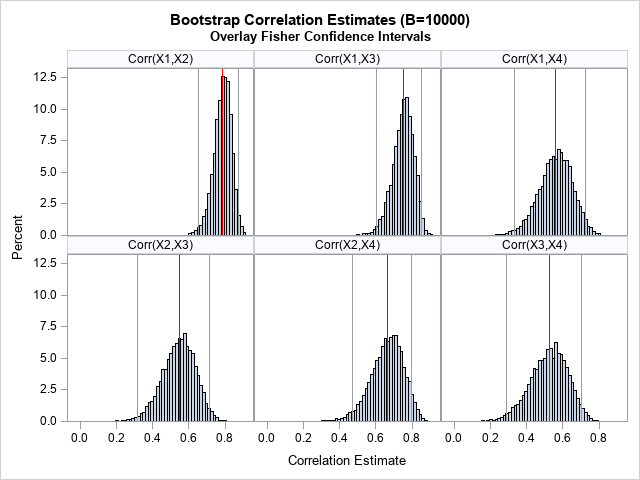You can use the bootstrap method to estimate confidence intervals. Unlike formulas, which assume that the data are drawn from a specified distribution (usually the normal distribution), the bootstrap method does not assume a distribution for the data. There are many articles about how to use SAS to bootstrap statistics for univariate data and for data from a regression model. This article shows how to bootstrap the correlation coefficients in multivariate data. The main challenge is that the correlation coefficients are typically output in a symmetric matrix. To analyze the bootstrap distribution, you must convert the statistics from a "wide form" (a matrix) to a "long form" (a column), as described in a previous article.

To motivate the bootstrap method for correlations, recall that PROC CORR in SAS supports the FISHER option, which estimates confidence intervals for the correlation coefficients. However, the interval estimates are parametric: they assume that the pairs of variables are bivariate normal. How good are the estimates for real data? You can generate the bootstrap distribution for the correlation coefficients and compare the Fisher confidence intervals to the bootstrap estimates. As a preview of things to come, the graph to the right (click to expand) shows histograms of bootstrap estimates and the Fisher confidence intervals.

This article assumes that the reader is familiar with basic bootstrapping in SAS. If not, see the article, "Compute a bootstrap confidence interval in SAS."

### Bootstrap multivariate data in SAS

The basic steps of the bootstrap algorithm are as follows:

1. Compute a statistic for the original data.
2. Use PROC SURVEYSELECT to resample (with replacement) B times from the data.
3. Use BY-group processing to compute the statistic of interest on each bootstrap sample.
4. Use the bootstrap distribution to obtain estimates of bias and uncertainty, such as confidence intervals.

Let's use a subset of Fisher's Iris data for this example. There are four variables to analyze, so you have to estimate confidence intervals for 4*3/2 = 6 correlation coefficients. For this example, let's use the FISHER option to estimate the confidence intervals. We can then compute the proportion of bootstrap estimates that fall within those confidence intervals, thereby estimating the coverage probability of the Fisher intervals? Does a 95% Fisher interval have 95% coverage for these data? Let's find out!

### Step 1: Compute statistics on the original data

The following SAS statements subset the Sashelp.Iris data and call PROC CORR to estimate Fisher's confidence intervals for the six correlation coefficients:

```data sample; /* N = 50 */ set Sashelp.Iris(where=(Species="Versicolor")); label PetalLength= PetalWidth= SepalLength= SepalWidth=; run;   /* STEP 1: Compute statistics on the original data */ %let varNames = PetalLength PetalWidth SepalLength SepalWidth; %let numVars=4; proc corr data=sample fisher(biasadj=no) noprob plots=matrix; var &varNames; ods output FisherPearsonCorr=FPCorr(keep=Var WithVar NObs Corr Lcl Ucl); run;   proc print data=FPCorr noobs; run;```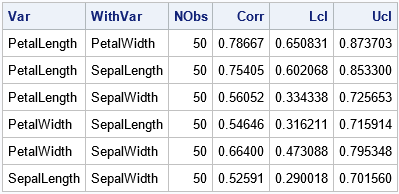The output shows the estimates for the 95% Fisher confidence intervals for the correlation coefficients. Remember, these estimates assume bivariate normality. Let's generate a bootstrap distribution for the correlation statistics and examine how well the Fisher intervals capture the sampling variation among the bootstrap estimates.

### Steps 2 and 3: Bootstrap samples and the bootstrap distribution

As shown in other articles, you can use PROC SURVEYSELECT in SAS to generate B bootstrap samples of the data. Each "bootstrap sample" is a sample (with replacement) from the original data. You can then use the BY statement in PROC CORR to obtain B correlation matrices, each estimated from one of the bootstrap samples:

```/* STEP 2: Create B=10,000 bootstrap samples */ /* Bootstrap samples */ %let NumSamples = 10000; /* number of bootstrap resamples */ proc surveyselect data=sample NOPRINT seed=12345 out=BootSamples method=urs /* resample with replacement */ samprate=1 /* each bootstrap sample has N observations */ /* OUTHITS option to suppress the frequency var */ reps=&NumSamples; /* generate NumSamples bootstrap resamples */ run;   /* STEP 3. Compute the statistic for each bootstrap sample */ proc corr data=BootSamples noprint outp=CorrOut; by Replicate; freq NumberHits; var &varNames; run;```

For many bootstrap analyses, you can proceed directly to the next step, which is to summarize the bootstrap distribution. However, in this case, the statistics in the CorrOut data set are not in a convenient format. The statistics are stored in matrices, as shown by the following call to PROC PRINT, which prints the correlation estimates for the first two bootstrap samples:

```/* look at estimates for first two bootstrap samples */ proc print data=CorrOut noobs; where _Type_="CORR" & Replicate<=2; run;```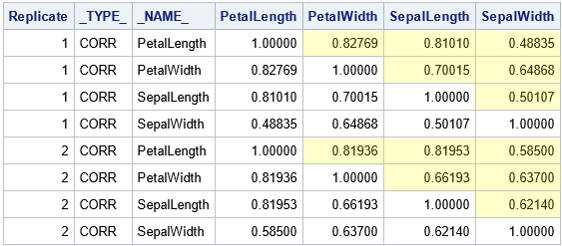To analyze the distribution of the correlation estimates, it is useful to reshape the data, as described in a previous article. The article shows how to obtain only the values in the cells that are highlighted in yellow. The article also shows how to add labels that identify the pairs of variables. The following DATA step reshapes the data and adds labels such as "Corr(X1,X2)":

```/* Convert correlations in the OUTP= data set from wide to long form. See https://blogs.sas.com/content/iml/2021/08/25/symmetric-matrix-wide-to-long.html */ data BootLong; set CorrOut(where=(_Type_="CORR") rename=(_NAME_=Var1)); length Var2 \$32 Label \$13; array X[*] &varNames; Row = 1 + mod(_N_-1, &NumVars); do Col = Row+1 to &NumVars; /* names for columns */ Var2 = vname(X[Col]); Corr = X[Col]; Label = cats("Corr(X",Row,",X",Col,")"); output; end; drop _TYPE_ &varNames; run;```

The bootstrap estimates are now in a "long format," which makes it easier to analyze and visualize the bootstrap distribution of the estimates.

The next section combines the original estimates and the bootstrap estimates. To facilitate combining the data sets, you need to add the Label column to the data set that contains the Fisher intervals. This was also described in the previous article. Having a common Label variable enables you to merge the two data sets.

```/* add labels to the Fisher statistics */ data FisherCorr; length Label \$13; set FPCorr; retain row col 1; if col>=&NumVars then do; row+1; col=row; end; col+1; Label = cats("Corr(X",row,",X",col,")"); run;```

### Step 4: Analyze the bootstrap distribution

You can graph the bootstrap distribution for each pair of variables by using the SGPANEL procedure. The following statements create a basic panel:

```proc sgpanel data=BootLong; panelby Label; histogram Corr; run;```

However, a goal of this analysis is to compare the Fisher confidence intervals (CIs) to the bootstrap distribution of the correlation estimates. Therefore, it would be nice to overlay the original correlation estimates and Fisher intervals on the histograms of the bootstrap distributions. To do that, you can concatenate the BootLong and the FisherCorr data sets, as follows:

```/* STEP 4: Analyze the bootstrap distribution */ /* overlay the original sample estimates and the Fisher confidence intervals */ data BootLongCI; set BootLong FisherCorr(rename=(Var=Var1 WithVar=Var2 Corr=Estimate)); label Corr="Correlation Estimate"; run;   title "Bootstrap Correlation Estimates (B=&NumSamples)"; title2 "Overlay Fisher Confidence Intervals"; proc sgpanel data=BootLongCI; panelby Label / novarname; histogram Corr; refline Estimate / axis=X lineattrs=(color=red); refline LCL / axis=X ; refline UCL / axis=X ; run;```

The graph is shown at the top of this article. In the graph, the red lines indicate the original estimates of correlation from the data. The gray vertical lines show the Fisher CIs for the data. The histograms show the distribution of the correlation estimates on 10,000 bootstrap re-samples of the data.

Visually, it looks like the Fisher confidence intervals do a good job of estimating the variation in the correlation estimates. It looks like most of the bootstrap estimates are contained in the 95% CIs.

### The coverage of Fisher's confidence intervals

You can use the bootstrap estimates to compute the empirical coverage probability of the Fisher CIs. That is, for each pair of variables, what proportion of the bootstrap estimates (out of 10,000) are within the Fisher intervals? If all pairs of variables were bivariate normal, the expected proportion would be 95%.

You can use the logical expression (Corr>=LCL & Corr<=UCL) to create a binary indicator variable. The proportion of 1s for this variable equals the proportion of bootstrap estimates that are inside a Fisher interval. The following DATA step creates the indicator variable and the call to PROC FREQ counts the proportion of bootstrap estimates that are in the Fisher interval.

```/* how many bootstrap estimates are inside a Fisher interval? */ proc sort data=BootLong; by Label; run;   data Pctl; merge BootLong FisherCorr(rename=(Corr=Estimate)); by Label; label Corr="Correlation Estimate"; InLimits = (Corr>=LCL & Corr<=UCL); run;   proc freq data=Pctl noprint; by Label; tables InLimits / nocum binomial(level='1'); output out=Est binomial; run; proc print data=Est noobs; var Label N _BIN_ L_BIN U_BIN; run;```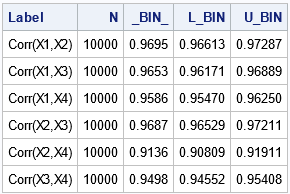The table shows empirical estimates for the coverage probability of the Fisher intervals. The _BIN_ column contains estimates of the binary proportion. The L_BIN and U_BIN columns contain confidence intervals for the proportions. You can see that all intervals include more than 90% of the bootstrap estimates, and some are close to 95% coverage. The following graph visualizes the empirical coverage for each pair of variables:

```ods graphics / width=400px height=240px; title "Proportion of Bootstrap Estimates in Fisher Intervals"; proc sgplot data=Est noautolegend; scatter x=_BIN_ y=Label / xerrorlower=L_Bin xerrorupper=U_Bin; refline 0.95 / axis=x; xaxis max=1 grid; yaxis grid display=(nolabel) offsetmin=0.1 offsetmax=0.1; run;```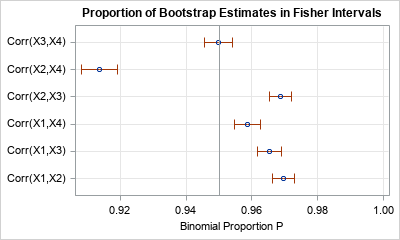The graph indicates that the many Fisher intervals are too wide (too conservative). Their empirical coverage is greater than the 95% coverage that you would expect for bivariate normal data. On the other hand, the Fisher interval for Corr(X2,X4) has lower coverage than expected.

### Summary

This article shows how to perform a bootstrap analysis for correlation among multiple variables. The process of resampling the data (PROC SURVEYSELECT) and generating the bootstrap estimates (PROC CORR with a BY statement) is straightforward. However, to analyze the bootstrap distributions, you need to restructure the statistics by converting the output data from a wide form to a long form. You can use this technique to perform your own bootstrap analysis of correlation coefficients.

Part of the analysis also used the bootstrap distributions to estimate the coverage probability of the Fisher confidence intervals for these data. The Fisher intervals have 95% coverage for normally distributed data. For the iris data, most of the intervals have coverage that is greater than expected, although one interval has lower-than-expected coverage. These results are strongly dependent on the data.

The post Bootstrap correlation coefficients in SAS appeared first on The DO Loop.For graphing multivariate data, it is important to be able to convert the data between "wide form" (a separate column for each variable) and "long form" (which contains an indicator variable that assigns a group to each observation). If the data are numeric, the wide data can be represented as an N x p matrix. The same data in long form can be represented by two columns and Np rows, where the first column contains the data and the second column identifies that the first N rows belong to the first group, the second N rows belong to the second group, and so on. Many people have written about how to use PROC TRANSPOSE or the SAS DATA step to convert from wide form to long form and vice versa.

The conversion is slightly different for a symmetric matrix because you might want to display only the upper-triangular portion of the matrix. This article examines how to convert between a symmetric correlation matrix (wide form) and a "compressed symmetric" form that only stores the elements in the upper-triangular portion of the symmetric matrix.

### Compressed symmetric storage

When the data represents a symmetric N x N matrix, you can save space by storing only half the data, such as only the upper triangular portion of the matrix. This is called compressed symmetric storage. Familiar examples of symmetric matrices include correlation, covariance, and distance matrices. For example, you can represent the 3 x 3 symmetric matrix A = {3 2 1, 2 5 0, 1 0 9} by storing only the upper triangular entries U = {3, 2, 1, 5, 0, 9}. There are N(N+1)/2 upper triangular elements. For a correlation matrix, you don't need to store the diagonal elements; you only need to store N(N-1)/2 elements.

When you run a correlation analysis by using PROC CORR in SAS, you usually get a square symmetric matrix of correlation estimates. However, if you use the FISHER option to get confidence limits for the correlation coefficients, then you get a table that shows the correlation estimates for each pair of variables. I will demonstrate this point by using the Sashelp.Iris data. To make the discussion general, I will put the variable names into a macro variable so that you can concentrate on the main ideas:

```data sample; /* use a subset of the Iris data */ set Sashelp.Iris(where=(Species="Versicolor")); label PetalLength= PetalWidth= SepalLength= SepalWidth=; run;   %let varNames = PetalLength PetalWidth SepalLength SepalWidth; /* count how many variables are in the macro variable */ data _null_; nv = countw("&varNames"); call symputx("numVars", nv); run; %put &=numVars; /* for this example, numVars=4 */   proc corr data=sample fisher(rho0=0.7 biasadj=no) noprob outp=CorrOut; var &varNames; ods select PearsonCorr FisherPearsonCorr; ods output FisherPearsonCorr = FisherPCorr; run;```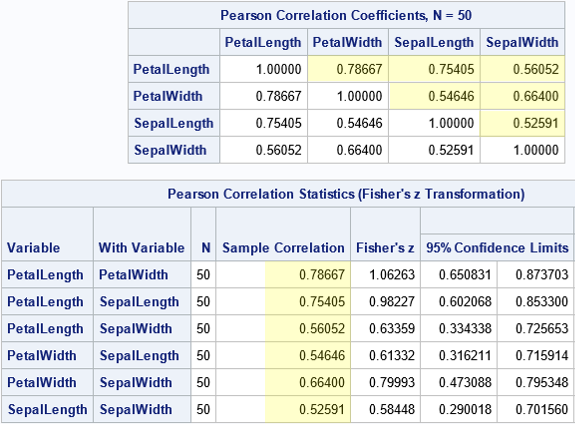The usual square symmetric correlation matrix is shown at the top of the image. I have highlighted the elements in the strictly upper triangular portion of the matrix. By using these values, you can re-create the entire matrix. The second table is an alternative display that shows only the six pairwise correlation estimates. The arrangement in the second table can be useful for plotting the pairwise correlations in a bar chart.

It is useful to be able to convert the first table into the format of the second table. It is also useful to be able to augment the second table with row/column information so that each row of the second table is easily associated with the corresponding row and column in the first table.

### Convert a symmetric table to a pairwise list

Let's use the information in the first table to list the correlations for pairs of variables, as shown in the second table. Notice that the call to PROC CORR uses the OUTP= option to write the correlation estimates to a SAS data set. You should print that data set to understand its structure; it contains more than just the correlation estimates! After you understand the structure, the following DATA step will make more sense. The main steps of the transformation are:

• Create a variable named ROW that has the values 1, 2, 3, ..., N. In the following DATA step, I use the MOD function because in a subsequent article, I will use the same DATA step to perform a bootstrap computation in which the data set contains B copies of the correlation matrix.
• Create a variable named COL. For each value of ROW, the COL variable loops over the values ROW+1, ROW+2, ..., N.
• Put the correlation estimates into a single variable named CORR.
• If desired, create a short label that identifies the two variables that produced the correlation estimate.
```/* Convert correlations in the OUTP= data set from wide to long form (pairwise statistics) */ data Long; set CorrOut(where=(_Type_="CORR") rename=(_NAME_=Var1)); length Var2 \$32 Label \$13; array X[*] &varNames; row = 1 + mod(_N_-1, &NumVars); /* 1, 2, ..., N, 1, 2, ..., N, 1, 2, ... */ do col = row+1 to &NumVars; /* names for columns */ Var2 = vname(X[col]); Corr = X[col]; Label = cats("Corr(X",row,",X",col,")"); output; end; drop _TYPE_ &varNames; run;   proc print data=Long; run;```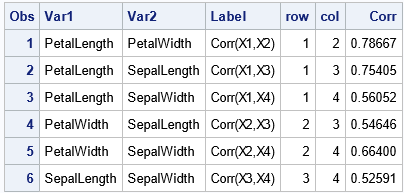The ROW and COL variables identify the position of the correlation estimates in the upper-triangular portion of the symmetric correlation matrix. You can write a similar DATA step to convert a symmetric matrix (such as a covariance matrix) in which you also want to display the diagonal elements.

### Identify the rows and columns of a pairwise list

The data for the second table is stored in the FisherPCorr data set. Although it is possible to convert the pairwise list into a dense symmetric matrix, a more useful task is to identify the rows and columns for each entry in the pairwise list, as follows:

```/* Write the (row,col) info for the list of pairwise correlations in long form */ data FisherCorr; set FisherPCorr; retain row col 1; if col>=&NumVars then do; row+1; col=row; end; col+1; Label = cats("Corr(X",row,",X",col,")"); run;   proc print data=FisherCorr; var Var WithVar Corr row col Label; run;```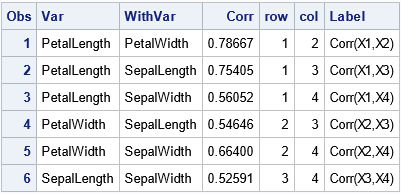From the ROW and COL variables, you can assemble the data into a symmetric matrix. For example, I've written about how to use the SAS/IML language to create a symmetric correlation matrix from the strictly upper-triangular estimates.

### Summary

For a symmetric matrix, you can display the matrix in a wide format (an N x N matrix) or you can display the upper-triangular portion of the matrix in a single column (long format). This article shows how to use the SAS DATA step to convert a correlation matrix into a long format. By adding some additional variables, you can identify each value with a row and column in the upper-triangular portion of the symmetric matrix.

The post Convert a symmetric matrix from wide to long form appeared first on The DO Loop.One of the benefits of using the SWEEP operator is that it enables you to "sweep in" columns (add effects to a model) in any order. This article shows that if you use the SWEEP operator, you can compute a SSCP matrix and use it repeatedly to estimate any linear regression model that uses those effects.

This post relates to a previous post, in which I showed that you never need to use a permutation matrix to rearrange the order of rows and columns of a matrix. As an application, I used the sum of squares and crossproducts (SSCP) matrix, which is used to estimate the regression coefficients in a least-squares regression model. Being able to permute the rows and columns of the SSCP matrix efficiently means that you can solve the linear regression problem very quickly for any ordering of the columns of the design matrix. But if you use the SWEEP operator, you do not need to permute the SSCP matrix at all!

### The SSCP matrix in PROC REG

Before discussing the SWEEP operator, let's review a little-used feature of PROC REG in SAS. The REG procedure is interactive, which means that you can interactively add or delete effects from a model as part of the model-building process. However, if you are going to explore several different models, you must use the VAR statement to specify all variables that you might conceivably want to use BEFORE the first RUN statement. Why? Because when PROC REG encounters the RUN statement it builds the SSCP matrix for the variables that you have specified. As stated in the documentation, for each subsequent model, "the procedure selects the appropriate crossproducts from the [SSCP] matrix." In other words, PROC REG re-uses that SSCP matrix for every MODEL statement.

Let's look at an example. The following call to PROC REG computes the SSCP matrix for five variables and the intercept effect. It uses that SSCP matrix to compute the parameter estimates:

```proc reg data=sashelp.cars plots=NONE; ods select ParameterEstimates; VAR MSRP EngineSize Horsepower MPG_Highway Weight; /* vars in the SSCP */ FULL: model MSRP = EngineSize Horsepower MPG_Highway Weight; /* uses the SSCP */ run;```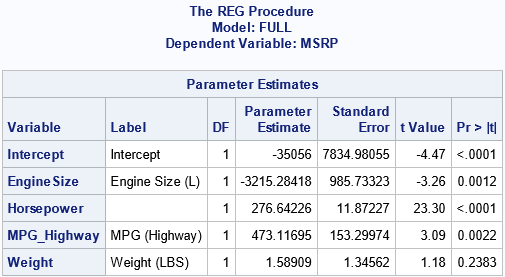Because PROC REG is an interactive procedure, the procedure does not exit when it encounters the RUN statement. It remembers the SSCP matrix until the procedure exits. If you submit additional MODEL statements, PROC REG will use the SSCP matrix to estimate the new parameters, as long as the variables were previously specified. For example, you can specify the same model, but list the variables in a different order. Or you can estimate a reduced model that contains only a subset of the variables, as follows:

``` PERMUTE: model MSRP = Horsepower Weight EngineSize MPG_Highway; run; PARTIAL: model MSRP = Weight Horsepower; run;quit;```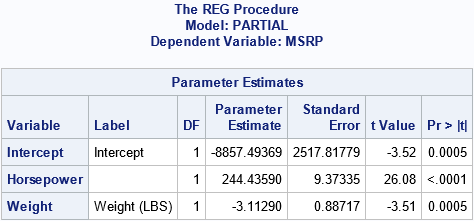The output for the "PERMUTE" model is not shown, because the estimates are the same as for the "FULL" model, but in a different order. It is important to note that PROC REG estimates the second and third models without re-reading the data. It simply re-uses the original SSCP matrix. As I have previously shown, computing the SSCP matrix is often 90% of the work of computing regression estimates, which means that the second and third models are computed very quickly.

The next section shows that how the SWEEP operator can quickly compute the parameter estimates for any model and for any order of the variables. There is no need to physically permute the rows and columns of the SSCP matrix.

### The SWEEP operator

You can use the SAS/IML language to illuminate how PROC REG can compute one SSCP matrix and re-use it for all subsequent models. The following SAS/IML statements read the data and compute the SSCP matrix. Following Goodnight (1979), the last column contains the response variable, but this is merely a convenience.

```proc iml; varNames = {'EngineSize' 'Horsepower' 'MPG_Highway' 'Weight'}; use sashelp.cars; read all var varNames into X; read all var 'MSRP' into Y; close; /* add intercept column and response variable */ X = j(nrow(X), 1, 1) || X; /* the design matrix */ k = ncol(X); /* number of effects */   varNames = 'Intercept' || varNames || 'MSRP'; XY = X || Y; SSCP = XY` * XY; /* the only SSCP that we'll need */   /* by using the sweep operator (1st row=mean, last col=param est*/ S = sweep(SSCP, 1:k); rCol = nrow(SSCP); /* the response column */ b = S[1:k, rCol]; /* parameter estimates for regression coefficients */ print b[r=varNames];```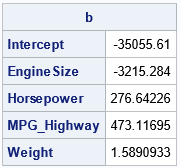The parameter estimates are the same as computed by PROC REG. They were obtained by sweeping the columns of the SSCP matrix in the order 1, 2, ..., 5. You can think of the vector v=1:k as the "identity permutation" of the rows. Then the SWEEP operator for the variables in their original order is the operation S = sweep(SSCP, v).

The SWEEP function in SAS/IML enables you to specify any vector of indices as the second argument. In this way, you can sweep the columns of the SSCP matrix in any order. For example, the "PERMUTE" model from the PROC REG example corresponds to sweeping the SSCP matrix in the order v = {1 3 5 2 4}. As the following example shows, you get the same parameter estimates because the models are the same:

```/* sweep original SSCP in the order of v */ v = {1 3 5 2 4}; pVarNames = varNames[,v]; /* effect names in permuted order */ S = sweep(SSCP, v); /* sweep the specified rows in the specified order */ b = S[v, rCol]; /* get the parameter estimates in new order */ print b[r=pVarNames];```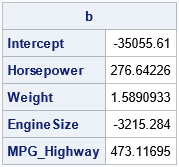As expected, the parameter estimates are the same, but the order of the parameters is different.

You can also estimate parameters for any model that uses a subset of the columns. For example, the model that has the explanatory variables Weight and Horsepower (the fifth and third effects) is computed from the SSCP matrix by sweeping the columns in the order {1 5 3}, as follows:

```/* Perform a partial sweep for the model {1 5 3}. No need to form a new design matrix or to extract a portion of the SCCP. */ v = {1 5 3}; pVarNames = varNames[,v]; /* effect names for this model */ S = sweep(SSCP, v); /* sweeps in the variables in the order of v */ b = S[v, rCol]; /* get the parameter estimates */ print b[r=pVarNames];```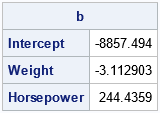The output matches the "PARTIAL" model from PROC REG.

### Summary

In a previous post, I showed an efficient way to permute the order of rows and columns of a matrix. But the current article shows that you do not need to permute the elements of an SSCP matrix if you use the SWEEP operator. The SWEEP operator enables you to specify the order in which you want to add effects to the model. From a design matrix, you can compute the SSCP matrix. (This is most of the work!) You can then quickly compute any model that uses the columns of the design matrix in any order.

This useful property of the SWEEP operator is among the many features discussed in Goodnight, J. (1979), "A Tutorial on the SWEEP Operator," The American Statistician. For other references, see the end of my previous article about the SWEEP operator.

The post More on the SWEEP operator for least-square regression models appeared first on The DO Loop.Do you ever use a permutation matrix to change the order of rows or columns in a matrix? Did you know that there is a more efficient way in matrix-oriented languages such as SAS/IML, MATLAB, and R? Remember the following tip:
Never multiply with a large permutation matrix! Instead, use subscripts to permute rows and columns.

This article explains why it is not necessary to multiply by a permutation matrix in a matrix language. The advice is similar to the tip in the article, "Never multiply with a large diagonal matrix," which discusses an efficient way to use diagonal matrices in matrix computations. That article recommends that you never multiply with a large diagonal matrix. Instead, use elementwise multiplication of rows and columns.

### Permutation matrices

Permutation matrices have many uses, but their primary use in statistics is to rearrange the order of rows or columns in a matrix. A previous article shows how to create a permutation matrix and how to use it to permute the order of rows and columns in a matrix. I ended the article by noting that "there is an easy alternative way" to permute rows and columns: use subscript notation. Subscripts enable you to permute rows and columns efficiently and intuitively. If you use matrix multiplication to permute columns of a matrix, you might not remember whether to multiply the permutation matrix on the left or on the right, but if you use subscripts, it is easy to remember the syntax.

The following example defines a 5 x 5 matrix, A, with integer entries and a function that creates a permutation matrix. (The permutation matrix is the transpose of the matrix that I used in the previous article. I think this definition is easier to use.) If you multiply A on the right (A*Q), you permute the columns. If you multiply A on the left (Q`*A), you permute the rows, as shown:

```proc iml; A = shape(1:25, 5, 5); /*{ 1 2 3 4 5, 6 7 8 9 10, ... 21 22 23 24 25} */ v = {3 5 2 4 1}; /* new order for the columns or rows */   /* Method 1: Explicitly form the permutation matrix */ /* the following matrix permutes the rows when P` is used on the left (P`*A) Use P on the right to permute columns. */ start PermutionMatrixT(v); return( I(ncol(v))[ ,v] ); finish; Q = PermutionMatrixT(v); C = A*Q; /* permute the columns */ R = Q`*A; /* permute the rows */ ods layout gridded advance=table columns=2; print R[r=v L="Permute Rows"], C[c=v L="Permute Columns"]; ods layout end;```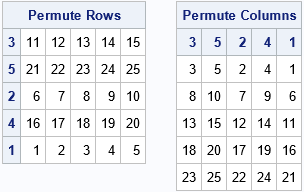This method explicitly forms a permutation matrix to permute the rows and/or columns. To permute the rows of an N x p matrix, the permutation matrix is an N x N matrix, and the matrix multiplication requires O(N2p) floating-point operations. To permute the columns, the permutation matrix is p x p, and the matrix multiplication requires O(Np2) operations.

### Permutation of indices for subscripts

In contrast, if you permute a matrix by using subscript indices, no floating-point operations are needed. The syntax A[v,] permutes the rows of the matrix A by using the indices in the vector v. The syntax A[,v] permutes the columns of A. The syntax A[v,v] permutes both the rows and the columns. Here is the previous permutation, but without using permutation matrices:

```/* Method 2: Specify subscript indices to form the permutation matrix */ C = A[ ,v]; /* permute the columns */ R = A[v, ]; /* permute the rows */ print R[r=v L="Permute Rows"], C[c=v L="Permute Columns"];```

The output is the same and is not shown. No computations are required, only copying data from one matrix to another. For v = {3 5 2 4 1}, the first syntax means, "copy the columns in the order, 3, 5, 2, 4, and 1." The second syntax specifies the order of the rows. You don't have to remember which side of A to put the permutation matrix, nor whether to use a transpose operation.

### An application: Order of effects in a regression model

In my previous article, I used a correlation matrix to demonstrate why it is useful to know how to permute rows and columns of a matrix. Another application is the order of columns in a design matrix for linear regression models. Suppose you read in a design matrix where the columns of the matrix are in a specified order. For some statistics (for example, the Type I sequential sum of squares), the order of the variables in the model are important. The order of variables is also used in regression techniques such as variable selection methods. Fortunately, if you have computed the sum of squares and crossproducts matrix (SSCP) for the variables in the original order, it is trivial to permute the matrix to accommodate any other ordering of the variables. If you have computed the SSCP matrix in one order, you can obtain it for any order without recomputing it.

The SSCP matrix is part of the "normal equations" that are used to obtain least-squares estimates for regression parameters. Let's look at an example. Suppose you compute the SSCP matrix for a data matrix that has five effects, as shown below:

```/* permute columns or rows of a SSCP matrix (XpX) */ use sashelp.cars; varNames = {'EngineSize' 'Horsepower' 'MPG_City' 'MPG_Highway' 'Weight'}; read all var varNames into Z; close;   X = Z - mean(Z); /* center the columns */ XpX = X`*X; print XpX[r=varNames c=varNames];```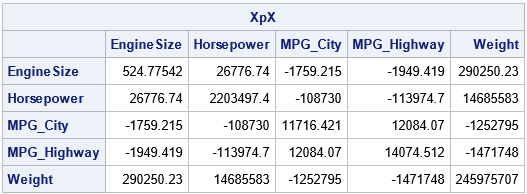Now, suppose you want the SSCP matrix for the same variables in a different order. For example, you want the order {'MPG_City' 'Weight' 'Horsepower' 'MPG_Highway' 'EngineSize'}; which is the permutation {3, 5, 2, 4, 1} of the original ordering. You have two choices: permute the columns of the design matrix and recompute the SSCP or permute the existing SSCP matrix. The first way is intuitive but less efficient. It is shown below:

```/* inefficient way: create new design matrix, Y, which permutes columns of X. Then compute SSCP. */ v = {3 5 2 4 1}; Q = PermutionMatrixT(v); Y = X*Q; sscp = Y`*Y; /* = Q`*X`*X*Q = Q`*(X`*X)*Q */ pVarNames = varNames[,v]; /* get new order for var names */ print sscp[r=pVarNames c=pVarNames];```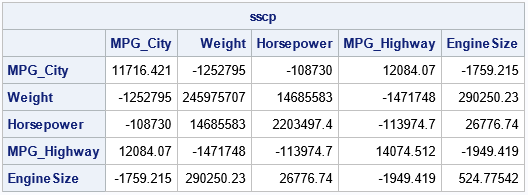The second way is a one-liner and does not require any computations. You don't have to form the permutation matrix, Q. You only need to use subscripts to permute the new SSCP:

```/* efficient way: don't form Q; just permute the indices */ sscp2 = XpX[v,v]; print sscp2[r=pVarNames c=pVarNames];```

The output is exactly the same and is not shown. After you have obtained the SSCP matrix in the new order, you can use it to compute regression statistics. If you are solving the normal equations, you also need to permute the right-hand side of the equations, which will be equal to Q`*X`*y.

### Summary

In summary, you rarely need to explicitly form a permutation matrix and use matrix multiplication to permute the rows or columns of a matrix. In most situations, you can use subscript indices to permute row, columns, or both rows and columns.

The post Never multiply with a large permutation matrix appeared first on The DO Loop.A SAS programmer recently asked why his SAS program and his colleague's R program display different estimates for the quantiles of a very small data set (less than 10 observations). I pointed the programmer to my article that compares the nine common definitions for sample quantiles. The article has a section that explicitly compares the default sample quantiles in SAS and R. The function in the article is written to support all nine definitions. The programmer asked whether I could provide a simpler function that computes only the default definition in R.

This article compares the default sample quantiles in SAS in R. It is a misnomer to refer to one definition as "the SAS method" and to another as "the R method." In SAS, procedures such as PROC UNIVARIATE and PROC MEANS enable you to use the QNTLDEF= to use five different quantile estimates. By using SAS/IML, you can compute all nine estimation methods. Similarly, R supports all nine definitions. However, users of statistical software often use the default methods, then wonder why they get different answers from different software. This article explains the difference between the DEFAULT method in SAS and the DEFAULT method in R. The default in R is also the default method in Julia and in the Python packages SciPy and NumPy.

### The Hyndman and Fan taxonomy

The purpose of a sample statistic is to estimate the corresponding population parameter. That is, the sample quantiles are data-based estimates of the unknown quantiles in the population. Hyndman and Fan ("Sample Quantiles in Statistical Packages," TAS, 1996), discuss nine definitions of sample quantiles that commonly appear in statistical software packages. All nine definitions result in valid estimates. For large data sets (say, 100 or more observations), they tend to give similar results. The differences between the definitions are most evident if you use a small data set that has wide gaps between two adjacent pairs of values (after sorting the data). The example in this article is small and has a wide gap between the largest value and the next largest value.

By default, SAS uses Hyndman and Fan's Type=2 method, whereas R (and Julia, SciPy, and NumPy) use the Type=7 method. The Type=2 method uses the empirical cumulative distribution of the data (empirical CDF) to estimate the quantiles, whereas the Type=7 method uses a piecewise-linear estimate of the cumulative distribution function. This is demonstrated in the next section.

### An example of sample quantiles

To focus the discussion, consider the data {0, 1, 1, 1, 2, 2, 2, 4, 5, 8}. There are 10 observations, but only six unique values. The following graphs show the estimates of the cumulative distribution function used by the Type=2 and Type=7 methods. The fringe plot (rug plot) below the CDF shows the locations of the data: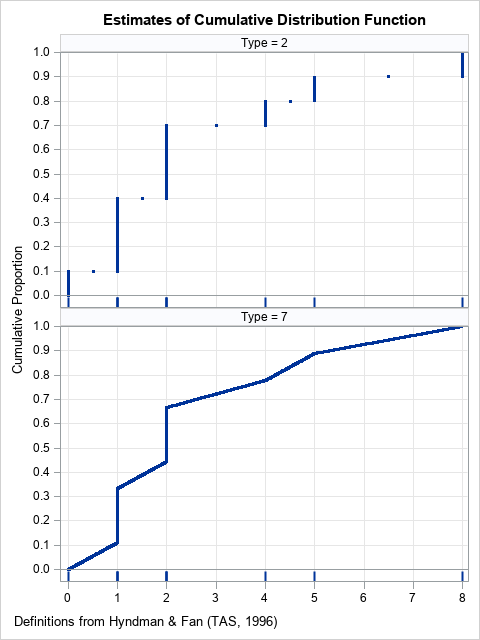The sample quantiles are determined by the estimates of the CDF. The largest gap in the data is between the values X=5 and X=8. So, for extreme quantiles (greater than 0.9), we expect to see differences between the Type=2 and the Type=7 estimates for extreme quantiles. The following examples show that the two methods agree for some quantiles, but not for others:

• The 0.5 quantile (the median) is determined by drawing a horizontal line at Y=0.5 and seeing where the horizontal line crosses the estimate of the CDF. For both graphs, the corresponding X value is X=2, which means that both methods give the same estimate (2) for the median.
• The 0.75 quantile (the 75th percentile) estimates are different between the two methods. In the upper graph, a horizontal line at 0.75 crosses the empirical CDF at X=4, which is a data value. In the lower graph, the estimate for the 0.75 quantile is X=3.5, which is neither a data value nor the average of adjacent values.
• The 0.95 quantile (the 95th percentile) estimates are different. In the upper graph, a horizontal line at 0.95 crosses the empirical CDF at X=8, which is the maximum data value. In the lower graph, the estimate for the 0.95 quantile is X=6.65, which is between the two largest data values.

### Comments on the CDF estimates

The Type=2 method (the default in SAS) uses an empirical CDF (ECDF) to estimate the population quantiles. The ECDF has a long history of being used for fitting and comparing distributions. For example, the Kolmogorov-Smirnov test uses the ECDF to compute nonparametric goodness-of-fit tests. When you use the ECDF, a quantile is always an observed data value or the average of two adjacent data values.

The Type=7 method (the default in R) uses a piecewise-linear estimate to the CDF. There are many ways to create a piecewise-linear estimate, and there have been many papers (going back to the 1930's) written about the advantages and disadvantages of each choice. In Hyndman and Fan's taxonomy, six of the nine methods use piecewise-linear estimates. Some people prefer the piecewise-linear estimates because the inverse CDF is continuous: a small change to the probability value (such as 0.9 to 0.91) results in a small change to the quantile estimates. This is property is not present in the methods that use the ECDF.

### A function to compute the default sample quantiles in R

Back in 2017, I wrote a SAS/IML function that can compute all of the common definitions of sample quantiles. If you only want to compute the default (Type=7) definition in R, you can use the following simpler function:

```proc iml; /* By default, R (and Julia, and some Python packages) uses Hyndman and Fan's Type=7 definition. Compute Type=7 sample quantiles. */ start GetQuantile7(y, probs); x = colvec(y); N = nrow(x); if N=1 then return (y); /* handle the degenerate case, N=1 */   /* remove missing values, if any */ idx = loc(x^=.); if ncol(idx)=0 then return (.); /* all values are missing */ else if ncol(idx)<N then do; x = x[idx,]; N = nrow(x); /* remove missing */ end;   /* Main computation: Compute Type=7 sample quantile. Estimate is a linear interpolation between x[j] and x[j+1]. */ call sort(x); p = colvec(probs); m = 1-p; j = floor(N*p + m); /* indices into sorted data values */ g = N*p + m - j; /* 0 <= g <= 1 for interpolation */   q = j(nrow(p), 1, x[N]); /* if p=1, estimate by x[N]=max(x) */ idx = loc(p < 1); if ncol(idx) >0 then do; j = j[idx]; g = g[idx]; q[idx] = (1-g)#x[j] + g#x[j+1]; /* linear interpolation */ end; return q; finish;   /* Compare the SAS and R default definitions. The differences between definitions are most apparent for small samples that have large gaps between adjacent data values. */ x = {0 1 1 1 2 2 2 4 5 8}`; prob = {0.5, 0.75, 0.9, 0.95};   call qntl(SASDefaultQntl, x, prob); RDefaultQntl = GetQuantile7(x, prob); print prob SASDefaultQntl RDefaultQntl;```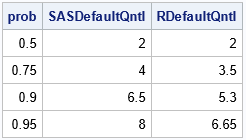The table shows some of the quantiles that were discussed previously. If you choose prob to be evenly spaced points in [0,1], you get the values on the graphs shown previously.

### Summary

There are many ways to estimate quantiles. Hyndman and Fan (1996) list nine common definitions. By default, SAS uses the Type=2 method, where R (and other software) uses the Type=7 method. SAS procedures support five of the nine common definitions of sample quantiles, and you can use SAS/IML to compute the remaining definitions. To make it easy to reproduce the default values of sample quantiles from other software, I have written a SAS/IML function that computes the Type=7 quantiles.

If you do not have SAS/IML software, but you want to compute estimates that are based on a piecewise-linear estimate of the CDF, I suggest you use the QNTLDEF=1 option in PROC UNIVARIATE or PROC MEANS. This produces the Type=4 method in Hyndman and Fan's taxonomy. For more information about the quantile definitions that are natively available in SAS procedures, see "Quantile definitions in SAS."

The post Compare the default definitions for sample quantiles in SAS, R, and Python appeared first on The DO Loop.To get better at something, you need to practice. That maxim applies to sports, music, and programming. If you want to be a better programmer, you need to write many programs. This article provides an example of forming the intersection of items in a SAS/IML list. It then provides several exercises so that SAS/IML programmers can practice working with lists.

### What are SAS/IML lists?

A SAS/IML list is a data structure that enables you to pack various SAS/IML objects (scalars, vectors, matrices, tables, ...) into a single object. A primary use of lists is to pass information between functions. You can create lists and extract items from lists by using a special syntax. For example, if L is a list then L\$1 is the first item in the list, L\$2 is the second item, and so on.

Recently, a SAS/IML programmer asked some basic questions about manipulating items in a list. The chapter about lists in the SAS/IML User's Guide includes many examples, but there is a big difference between reading documentation and writing a program yourself. This article provides exercises that enable you to practice working with lists.

### The intersection of items in a list

This section shows how to compute the intersection of items in a list. The input is a SAS/IML list that contains many matrices. The output is the intersection between the items.

If you have a set of matrices, the XSECT function in SAS/IML computes the intersection of the values in the matrices. However, you cannot pass a list to the XSECT function. The following example shows that the XSECT function takes a comma-separated set of matrices:

```proc iml; x = 1:20; /* 1,2,3,...,20 */ y = do(0, 20, 2); /* 0,2,4,...,20 */ z = do(0, 21, 3); /* 0,3,6,...,21 */ w = 3:24; /* 3,4,5,...,24 */   intersect = xsect(x,y,z,w); print intersect;```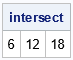The intersection of the matrices (thought of as sets) contains three elements. Notice that the arguments to the XSECT function are matrices.

So, how could you compute the intersection of matrices if they are items in a list? The key is to recognize that the intersection of k sets is equal to taking k-1 intersections. First, form S1 as the intersection of X and Y. Then form S2 as the intersection of S1 and Z. Lastly, form S3 (the answer) as the intersection of S2 and W. Thus, you can compute the total intersection by always computing the intersection of pairs of sets.

Thus, you can compute the intersection by looping over the items in the list. You can use the ListLen function to get the number of items in a list. Then repeatedly compute the pairwise intersections, as follows:

```start ListIntersect(L); len = ListLen(L); if len=0 then /* no items in list */ return ( {} ); /* return empty matrix */ else if len=1 then /* one item */ return L\$1; /* return the item */   intersect = xsect(L\$1, L\$2); /* the intersection of the first 2 items */ do i = 3 to len; intersect = xsect(intersect, L\$i); /* the intersection of the first i items */ end; return intersect; finish;   /* test the function: pack matrices into a list */ L = [x, y, z, w]; s = ListIntersect(L); print s;```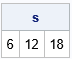Success! The ListIntersect function returns the same values as the original call to the XSECT function.

### Exercises for manipulating lists

1. The previous example shows that the ListIntersection function works on a list that contains numerical matrices. But the XSECT function also works for character values. Use the following character matrices to test whether the ListIntersect function works for a list that contains character items. If not, modify the function so that it works for a list of character matrices.
```a = 'A':'Z'; b = {A C E G I K M O Q S U W Y}; c = {A B C F G I K N O R S V Y}; d = {I THINK IT IS EITHER X OR Y};```
2. The ListIntersect function does not check that the items in the list are the same type. Use the TYPE function to ensure that all items in the list have the same type, either all numeric or all character. If not, return the empty matrix.
3. Write a function called ListUnion that uses the UNION function to compute the union of the elements in a list. Make sure it handles lists that contain all numeric or all character items.
4. Given a matrix (m) and a list (L), you can check to see which items in the list have a nonempty intersection with the matrix. If there are N items in the list, write a function called HasIntersection that returns a binary vector (v) of length N. The i_th value, v[i], is 1 if XSECT(m, L\$i) is nonempty. Otherwise, v[i]is 0. For example, the following call should return the vector {0 1 1 1} because L has four items and m has a nonempty intersection with items 2, 3, and 4:
```m = {0 24}; v = HasIntersection(m, L);```

### Summary

If you want to be good at something, you need to practice. This article shows how to manipulate items in a SAS/IML list to form the intersection of items. It then provides a set of exercises that a motivated programmer can use to practice working with lists.

The post Operations on lists in SAS/IML appeared first on The DO Loop.After my recent articles on simulating data by using copulas, many readers commented about the power of copulas. Yes, they are powerful, and the geometry of copulas is beautiful. However, it is important to be aware of the limitations of copulas. This article creates a bizarre example of bivariate data, then shows what happens when you fit copula models to the example.

You might think that if you match the correlation and marginal distributions of data, your model must look similar to the data. However, that statement is false. This article demonstrates that there are many bivariate distributions that have the same correlation and marginal distributions. Some might look like the data; others do not. To keep the discussion as simple as possible, I will discuss only bivariate data with marginal distributions that are normal.

### Many ways to generate normally distributed variables

Before constructing the bizarre bivariate example, let's look at an interesting fact about symmetric univariate distributions. Let Z ~ N(0,1) be a standard normal random variable. Consider the following methods for generating a new random variable from Z:

• Y = Z: Clearly, Y is a standard normal random variable.
• Y = –Z: Since Z is symmetric, Y is a standard normal random variable.
• With probability p, Y = –Z; otherwise, Y = Z: This is a little harder to understand, but Y is still a normal random variable! Think about generating a large sample from Z. Some observations are negative, and some are positive. To get a sample from Y, you randomly change the sign of each observation with probability p. Because the distribution is symmetric, you change half the positives to negatives and half the negatives to positives. Thus, Y is normally distributed. Both previous methods are special cases of this one. If p=0, then Y = Z. If p=1, then Y = –Z.

To demonstrate the third method, the following SAS DATA step generates a normally distributed sample by generating Z~N(0,1), then randomly permuting the sign of 50% of the variates:

```%let N = 2000; data Sim; call streaminit(1234); p = 0.5; do i = 1 to &N; z = rand("Normal"); /* flip the sign of Z with probability p */ if rand("Bernoulli", p) then y = z; else y = -z; output; end; run;   proc univariate data=Sim; var y; histogram / normal; run;```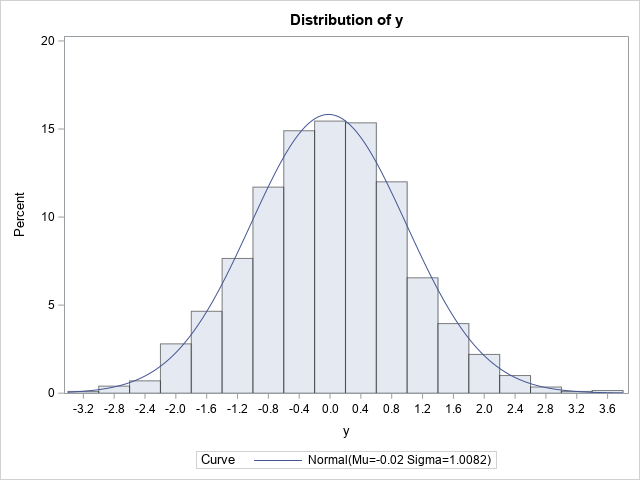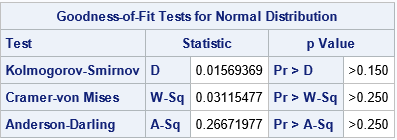The graph indicates that Y is normally distributed. If you prefer statistical tests, the table shows several goodness-of-fit tests. The results indicate that a normal distribution fits the simulated data well.

This example shows that you can change the signs of 50% of the observations and still obtain a normal distribution. This fact is used in the next section to construct a bizarre bivariate distribution that has normal marginals. (Note: Actually, this example is valid for any value of p, 0 ≤ p ≤ 1. You always get normally distributed data.)

### A bizarre example: Normal marginals do not imply bivariate normality

Let's play a game. I tell you that I have bivariate data where each variable is normally distributed and the correlation between the variables is 0.75. I ask you to sketch what you think a scatter plot of my data looks like. Probably most people would guess it looks like the graph below, which shows bivariate normal data: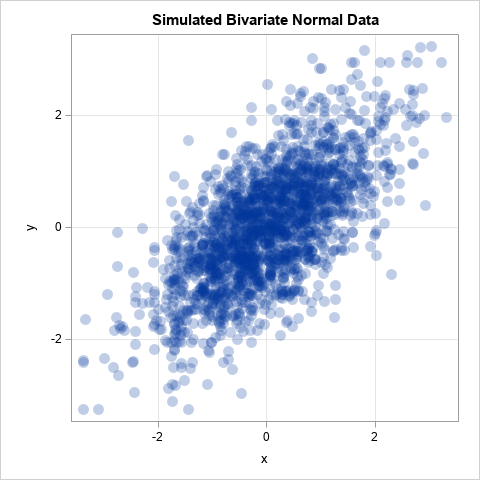If the joint distribution of X and Y is bivariate normal, then the univariate distributions of X and Y are indeed normal. However, the converse of that statement is not true! There are many bivariate distributions that are not bivariate normal, yet their marginal distributions are normal. Can't picture it? Study the following SAS DATA step, which simulates (X,Z) as bivariate data, but changes the sign for 50% of the Z variates:

```/* Bivariate data whose marginals are normal. Idea from https://stats.stackexchange.com/questions/30159/is-it-possible-to-have-a-pair-of-gaussian-random-variables-for-which-the-joint-d */ %let N = 2000; data NotBinormal; call streaminit(123); do i = 1 to &N; x = rand("Normal"); z = rand("Normal"); in24 = (x< 0 & z>=0) | /* (x,z) in 2nd quadrant */ (x>=0 & z< 0); /* (x,z) in 4th quadrant */ /* If (x,z) is in 2nd quadrant, flip into 3rd quadrant. If (x,z) is in 4th quadrant, flip into 1st quadrant. */ if in24 then y = -z; else y = z; output; end; run;   ods graphics / width=480px height=480px; proc corr data=NotBinormal noprob Spearman plots=matrix(hist); var x y; ods select SpearmanCorr MatrixPlot; run;```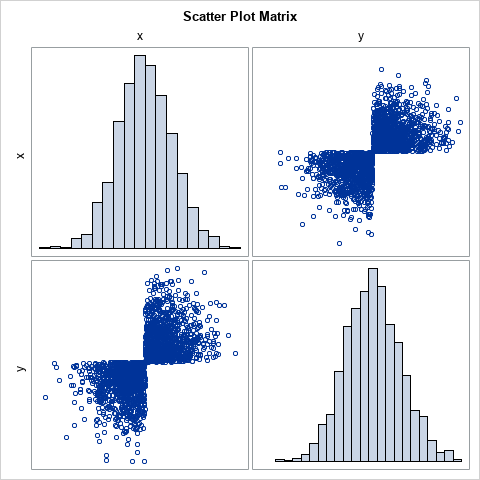The scatter plot looks like a bow tie! I saw this example on StackExchange in an answer by 'cardinal'. I like it because the joint distribution is obviously NOT bivariate normal even though X and Y are both standard normal variables. Obviously, the variable X is univariate normal. For the variable Y, notice that the program generates Z as random normal but changes the sign for a random 50% of the Z values. In this case, the 50% is determined by looking at the location of the (X,Z) points. If (X,Z) is in the second or fourth quadrants, which occurs with probability p=0.5, flip the sign of Z. The result is bivariate data that lies in only two quadrants. Yet, both marginal variables are normal.

When asked to describe the scatter plot of correlated data with normal marginals, I think that few people would suggest this example! Fortunately, real data does not look like this pathological example. Nevertheless, it is instructive to ask, "what does a copula model look like if we fit it to the bow-tie data?"

### Fit a copula to data

Let's fit a copula to the bizarre bow-tie distribution. We know from a previous article that a copula will have the same Spearman correlation (0.75) and the marginal distributions (normal) as the data. But if you simulate data from a Gaussian copula, a scatter plot of the simulated data looks nothing like the bow-tie scatter plot. Instead, you get bivariate normal data, as demonstrated by the following statements:

```proc copula data=NotBinormal; var x y; /* original data vars */ fit normal; /* choose copula: Normal, Clayton, Frank,... */ simulate / seed=1234 ndraws=&N marginals=empirical /* transform from copula by using empirical CDF of data */ out=SimData; /* contains the simulated data */ run;   title "Bivariate Normal Data"; proc sgplot data=SimData aspect=1; scatter x=x y=y / transparency=0.75 markerattrs=(symbol=CircleFilled size=12); xaxis grid; yaxis grid; run;```

The scatter plot is shown at the beginning of the previous section.

The COPULA procedure supports other types of copulas, such as the Clayton, Frank, and Gumbel copulas. These are useful in modeling data that have more (or less) weight in the tails, or that might be unsymmetric. For example, the Gumbel copula is an unsymmetric distribution that has more weight in the right tail than the normal distribution does. If you change the keyword NORMAL to GUMBEL in the previous call to PROC COPULA, you obtain the following scatter plot of simulated data from the Gumbel copula: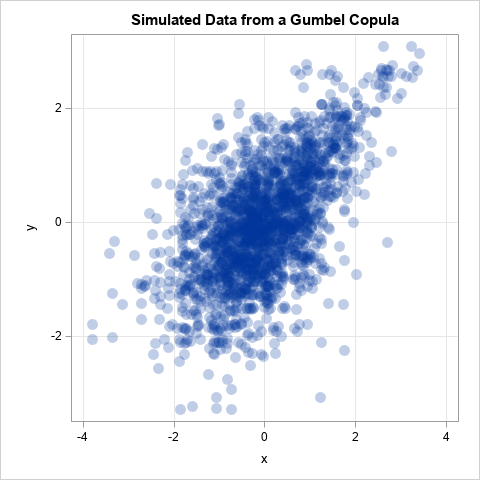This is another example of a distribution that has the same Spearman correlation as the data and has normal marginals. It is not bivariate normal, but it still doesn't look like the pathological bow-tie example.

### Summary

When you learn a new technique, it is important to know its limitations. This article demonstrates that there are many distributions that have the same correlation and the same marginal distributions. I showed a very dramatic example of a bizarre scatter plot that looks like a bow tie but, nevertheless, has normal marginals. When you fit a copula to the data, you preserve the Spearman correlation and the marginal distributions, but none of the copula models (Gaussian, Gumble, Frank, ....) look like the data.

I strongly encourage modelers to use the simulation capabilities of PROC COPULA to help visualize whether a copula model fits the data. You can make a scatter plot matrix of the marginal bivariate distributions to understand how the model might deviate from the data. As far as I know, PROC COPULA does not support any goodness-of-fit tests, so a visual comparison is currently the best you can do.

For more information about PROC COPULA in SAS, See the SAS/ETS documentation. (Or, for SAS Viya customers, see PROC CCOPULA in the SAS Econometrics product.)

• Venter, Gary G. (2002) "Tails of copulas," in Proceedings of the Casualty Actuarial Society (Vol. 89, No. 171, pp. 68-113).
• Zivot, Eric (to appear), "Copulas" in Modeling Financial Time Series with R, Springer-Verlag.

The post Copulas and multivariate distributions with normal marginals appeared first on The DO Loop.Do you know what a copula is? It is a popular way to simulate multivariate correlated data. The literature for copulas is mathematically formidable, but this article provides an intuitive introduction to copulas by describing the geometry of the transformations that are involved in the simulation process. Although there are several families of copulas, this article focuses on the Gaussian copula, which is the simplest to understand.

This article shows the geometry of copulas. This article and its example are based on Chapter 9 of Simulating Data with SAS (Wicklin, 2013). A previous article shows the geometry of the Iman-Conover transformation, which is an alternative way to create simulated data that have a specified rank correlation structure and specified marginal distributions.

### Simulate data by using a copula

Recall that you can use the CDF function to transform any distribution to the uniform distribution. Similarly, you can use the inverse CDF to transform the uniform distribution to any distribution. To simulate correlated multivariate data from a Gaussian copula, follow these three steps:

1. Simulate correlated multivariate normal data from a correlation matrix. The marginal distributions are all standard normal.
2. Use the standard normal CDF to transform the normal marginals to the uniform distribution.
3. Use inverse CDFs to transform the uniform marginals to whatever distributions you want.

The transformation in the second and third steps are performed on the individual columns of a data matrix. The transformations are monotonic, which means that they do not change the rank correlation between the columns. Thus, the final data has the same rank correlation as the multivariate normal data in the first step.

The next sections explore a two-dimensional example of using a Gaussian copula to simulate correlated data where one variable is Gamma-distributed and the other is Lognormally distributed. The article then provides intuition about what a copula is and how to visualize it. You can download the complete SAS program that generates the example and creates the graphs in this article.

### A motivating example

Suppose that you want to simulate data from a bivariate distribution that has the following properties:

• The rank correlation between the variables is approximately 0.6.
• The marginal distribution of the first variable, X1, is Gamma(4) with unit scale.
• The marginal distribution of the second variable, X2, is lognormal with parameters μ=0.5 and σ=0.8. That is, log(X2) ~ N(μ, σ).

The hard part of a multivariate simulation is getting the correlation structure correct, so let's start there. It seems daunting to generate a "Gamma-Lognormal distribution" with a correlation of 0.6, but it is straightforward to generate a bivariate NORMAL distribution with that correlation. Let's do that. Then we'll use a series of transformations to transform the normal marginal variables into the distributions that we want while preserving the rank correlation at each step.

### Simulate multivariate normal data

The SAS/IML language supports the RANDNORMAL function, which can generate multivariate normal samples, as shown in the following statements:

```proc iml; N = 1e4; call randseed(12345); /* 1. Z ~ MVN(0, Sigma) */ Sigma = {1.0 0.6, 0.6 1.0}; Z = RandNormal(N, {0,0}, Sigma); /* Z ~ MVN(0, Sigma) */```

The matrix Z contains 10,000 observations drawn from a bivariate normal distribution with correlation coefficient ρ=0.6. Because the mean vector is (0,0) and the covariance parameter is a correlation matrix, the marginal distributions are standard normal. The following graph shows a scatter plot of the bivariate normal data along with histograms for each marginal distribution.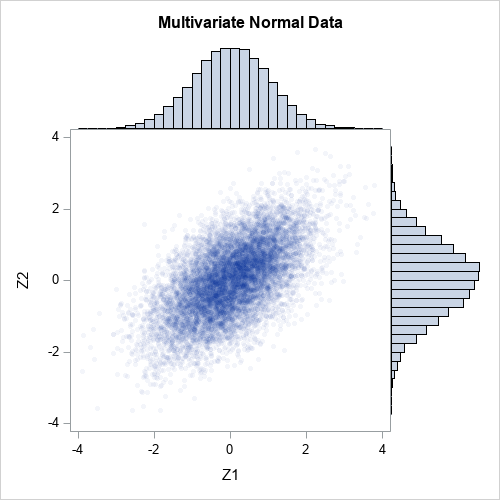### Transform marginal distributions to uniform

The first step is to transform the normal marginals into a uniform distribution by using the probability integral transform (also known as the CDF transformation). The columns of Z are standard normal, so Φ(X) ~ U(0,1), where Φ is the cumulative distribution function (CDF) for the univariate normal distribution. In SAS/IML, the CDF function applies the cumulative distribution function to all elements of a matrix, so the transformation of the columns of Z is a one-liner:

```/* 2. transform marginal variables to U(0,1) */ U = cdf("Normal", Z); /* U_i are correlated U(0,1) variates */```

The columns of U are samples from a standard uniform distribution. However, the columns are not independent. Because the normal CDF is a monotonic transformation, it does not change the rank correlation between the columns. That is, the rank correlation of U is the same as the rank correlation of Z:

```/* the rank correlations for Z and U are exactly the same */ rankCorrZ = corr(Z, "Spearman"); rankCorrU = corr(U, "Spearman"); print rankCorrZ rankCorrU;```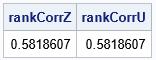The following graph shows a scatter plot of the transformed data along with histograms for each marginal distribution. The histograms show that the columns U1 and U2 are uniformly distributed on [0,1]. However, the joint distribution is correlated, as shown in the following scatter plot: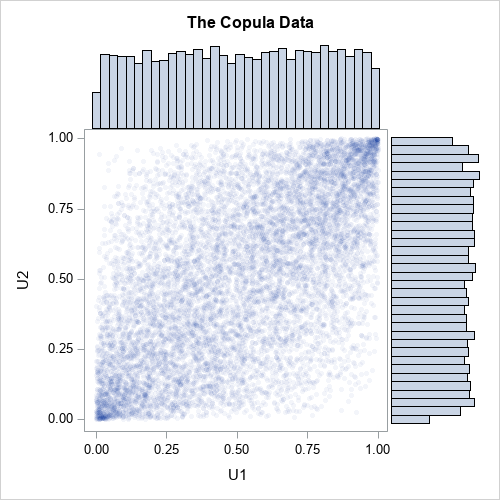### Transform marginal distributions to any distribution

Now comes the magic. One-dimensional uniform variates are useful because you can transform them into any distribution! How? Just apply the inverse cumulative distribution function of whatever distribution you want. For example, you can obtain gamma variates from the first column of U by applying the inverse gamma CDF. Similarly, you can obtain lognormal variates from the second column of U by applying the inverse lognormal CDF. In SAS, the QUANTILE function applies the inverse CDF, as follows:

```/* 3. construct the marginals however you wish */ gamma = quantile("Gamma", U[,1], 4); /* gamma ~ Gamma(alpha=4) */ LN = quantile("LogNormal", U[,2], 0.5, 0.8); /* LN ~ LogNormal(0.5, 0.8) */ X = gamma || LN;   /* check that the rank correlation still has not changed */ rankCorrX = corr(X, "Spearman"); print rankCorrZ rankCorrX;```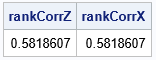The first column of X is gamma-distributed. The second column of X is lognormally distributed. But because the inverse of a continuous CDF is monotonic, the column transformations do not change the rank correlation between the columns. The following graph shows a scatter plot of the newly transformed data along with histograms for each marginal distribution. The histograms show that the columns X1 and X2 are distributed as gamma and lognormal, respectively. The joint distribution is correlated.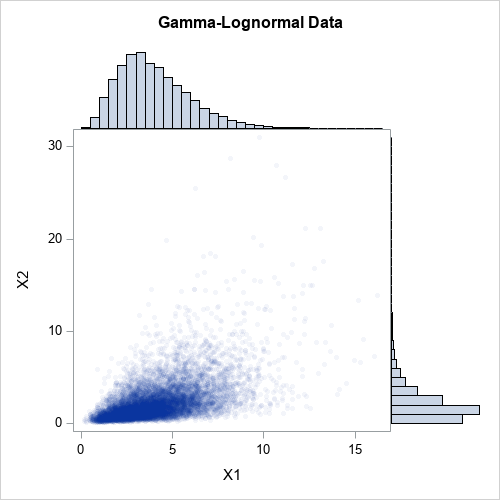### What about the Pearson correlation?

For multivariate normal data, the Pearson correlation is close to the rank correlation. However, that is not true for nonnormal distributions. The CDF and inverse-CDF transformations are nonlinear, so the Pearson correlation is not preserved when you transform the marginal. For example, the following statements compute the Pearson correlation for Z (the multivariate normal data) and for X (the gamma-lognormal data):

```rhoZ = corr(Z, "Pearson"); rhoX = corr(X, "Pearson"); print rhoZ rhoX;```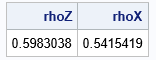Chapter 9 of Wicklin (2013), discusses the fact that you can adjust the correlation for Z and it will affect the correlation for X in a complicated manner. With a little work, you can choose a correlation for Z that will result in a specified correlation for X. For example, if you want the final Pearson correlation for X to be 0.6, you can use 0.642 as the correlation for Z:

```/* re-run the example with new correlation 0.642 */ Sigma = {1.0 0.642, 0.642 1.0}; newZ = RandNormal(N, {0,0}, Sigma); newU = cdf("Normal", newZ); /* columns of U are U(0,1) variates */ gamma = quantile("Gamma", newU[,1], 4); /* gamma ~ Gamma(alpha=4) */ expo = quantile("Expo", newU[,2]); /* expo ~ Exp(1) */ newX = gamma || expo;   rhoZ = corr(newZ, "Pearson"); rhoX = corr(newX, "Pearson"); print rhoZ rhoX;```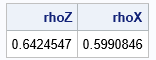Success! The Pearson correlation between the gamma and lognormal variables is 0.6. In general, this is a complicated process because the value of the initial correlation depends on the target value and on the specific forms of the marginal distributions. Finding the initial value requires finding the root of an equation that involves the CDF and inverse CDF.

### Higher dimensional data

This example in this article generalizes to higher-dimensional data in a natural way. The SAS program that generates the example in this article also includes a four-dimensional example of simulating correlated data. The program simulates four correlated variables whose marginal distributions are distributed as gamma, lognormal, exponential, and inverse Gaussian distributions. The following panel shows the bivariate scatter plots and marginal histograms for this four-dimensional simulated data.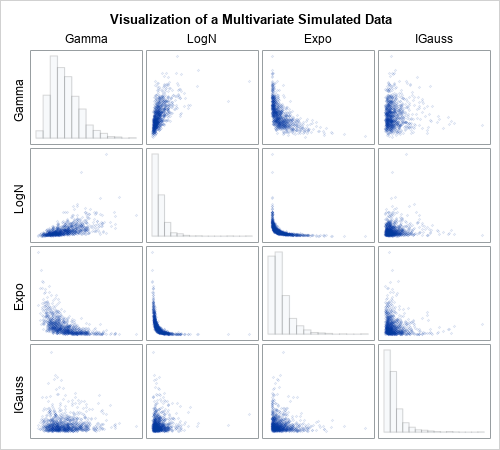### What is a copula?

I've shown many graphs, but what is a copula? The word copula comes from a Latin word (copulare) which means to bind, link, or join. The same Latin root gives us the word "copulate." A mathematical copula is a joint probability distribution that induces a specified correlation structure among independent marginal distributions. Thus, a copula links or joins individual univariate distributions into a joint multivariate distribution that has a specified correlation structure.

Mathematically, a copula is any multivariate cumulative distribution function for which each component variable has a uniform marginal distribution on the interval [0, 1]. That's it. A copula is a cumulative distribution function whose domain is the cube [0,1]d. So the second graph in this article is the graph most nearly related to the copula for the bivariate data, since it shows the relationship between the uniform marginals.

The scatter plot shows the density, not the cumulative distribution. However, you can use the data to estimate the bivariate CDF. The following heat map visualizes the Gaussian copula for the correlation of 0.6: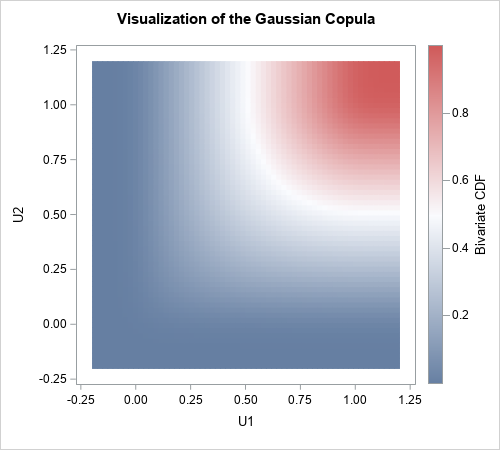Notice that this function does not depend on the final marginal distributions. It depends only on the multivariate normal distribution and the CDF transformation that produces the uniform marginals. In that sense, the copula captures only the correlation structure, without regard for the final form of the marginal distributions. You can use this same copula for ANY set of marginal distributions because the copula captures the correlation structure independently of the final transformations of the marginals.

### Why are copulas important?

A remarkable theorem (Sklar’s theorem) says that every joint distribution can be written as a copula and a set of marginal distributions. If the marginals are continuous, then the copula is unique. Let that sink in for a second: Every continuous multivariate probability distribution can be expressed in terms of its marginal distributions and a copula. Every one. So, if you can understand copulas you can understand all multivariate distributions. Powerful math, indeed!

For simulation studies, you can use the converse of Sklar's theorem, which is also true. Specifically, if you have a set of d uniform random variables and a set of marginal distribution functions, a copula transforms the d components into a d-dimensional probability distribution.

### Summary

Copulas are mathematically sophisticated. However, you can use copulas to simulate data without needing to understand all the mathematical details. This article presents an example of using a Gaussian copula to simulate multivariate correlated data. It shows the geometry at each step of the three-step process:

1. Simulate data from a multivariate normal distribution with a known correlation matrix.
2. Use the normal CDF to transform the marginal distributions to uniform.
3. Use inverse CDFs to obtain any marginal distributions that you want.

The result is simulated correlated multivariate data that has the same rank correlation as the original simulated data but has arbitrary marginal distributions.

This article uses SAS/IML to show the geometry of the copula transformations. However, SAS/ETS software provides PROC COPULA, which enables you to perform copula modeling and simulation in a single procedure. A subsequent article shows how to use PROC COPULA.

The post An introduction to simulating correlated data by using copulas appeared first on The DO Loop.